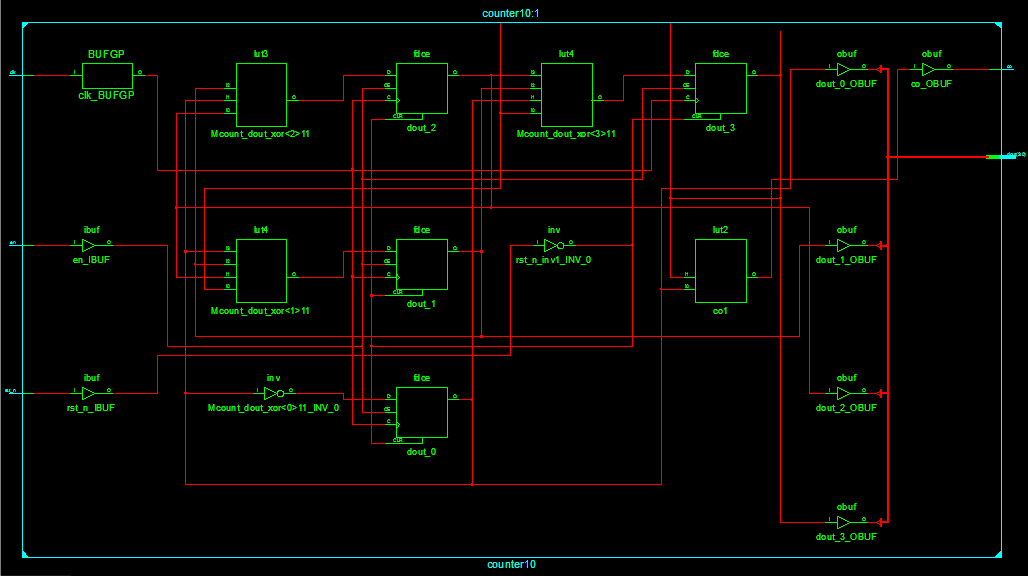# 模6计数器以及模10计数器（Verilog HDL语言设计）（Modelsim仿真与ISE综合）

• 时间:

Verilog HDL语言描述

RTL Schematic

Technology Schematic

Verilog HDL语言描述

RTL Schematic

Technology Schematic

## 模6计数器

### Verilog HDL语言描述

``````//模6计数器的Verilog HDL设计
module counter6(clk, rst_n, en, dout, co);

input clk, rst_n, en;
output[3:0] dout;
reg [3:0] dout;
output co;

always@(posedge clk or negedge rst_n)
begin
if(!rst_n)
dout <= 4'b0000;        //系统复位，计数器清零
else if(en)
if(dout == 4'b0101)     //计数值达到5时，计数器清零
dout <= 4'b0000;
else
dout <= dout + 1'b1; //否则，计数器加1
else
dout <= dout;

end

assign co = dout&dout;  //当计数达到5(4'b0101)时，进位为1，计数值为其他，都没有进位

endmodule
``````

### 测试文件

``````//模6计数器的测试文件
`timescale 1ns/1ps
module counter6_tb;

reg clk, rst_n, en;
wire[3:0] dout;
wire co;

//时钟设计周期为2ns
always
begin
#1 clk = ~clk;
end

//初始化
initial
begin
clk = 1'b0;
rst_n = 1'b1;
en = 1'b0;
#2 rst_n = 1'b0;
#2 rst_n = 1'b1; en = 1'b1;    //计数使能信号有效，且不复位

end

counter6 u1(.clk(clk), .rst_n(rst_n), .en(en), .dout(dout), .co(co));

endmodule

``````

### 仿真电路图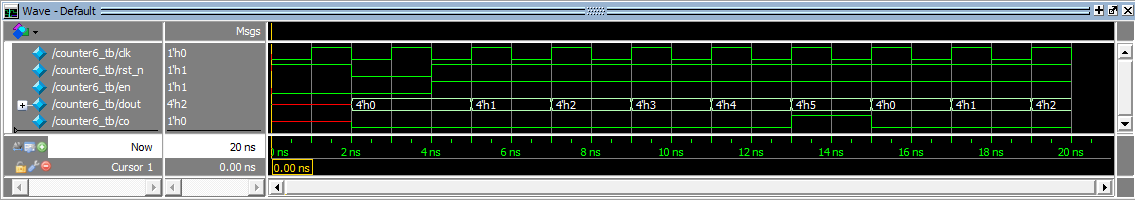### RTL Schematic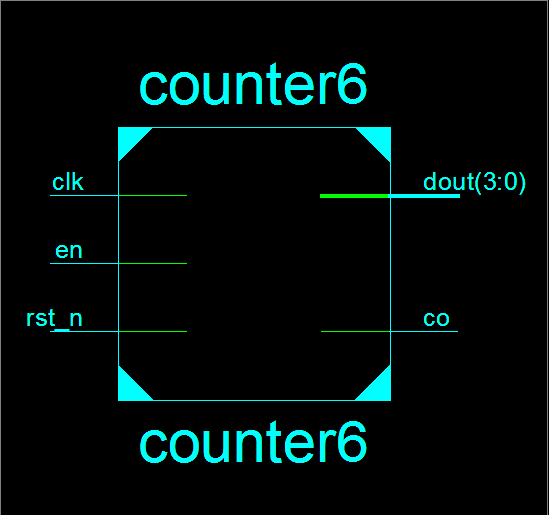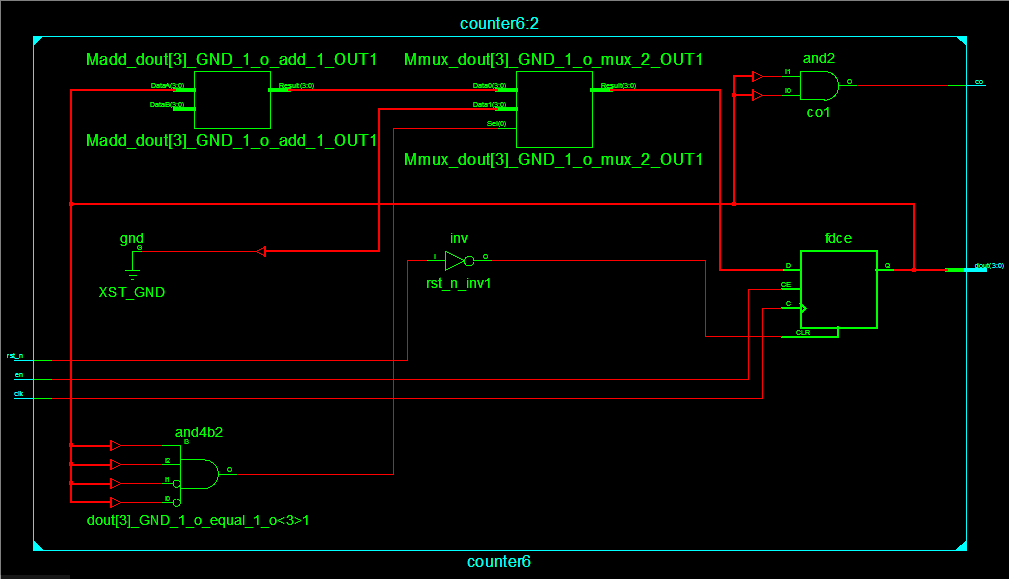### Technology Schematic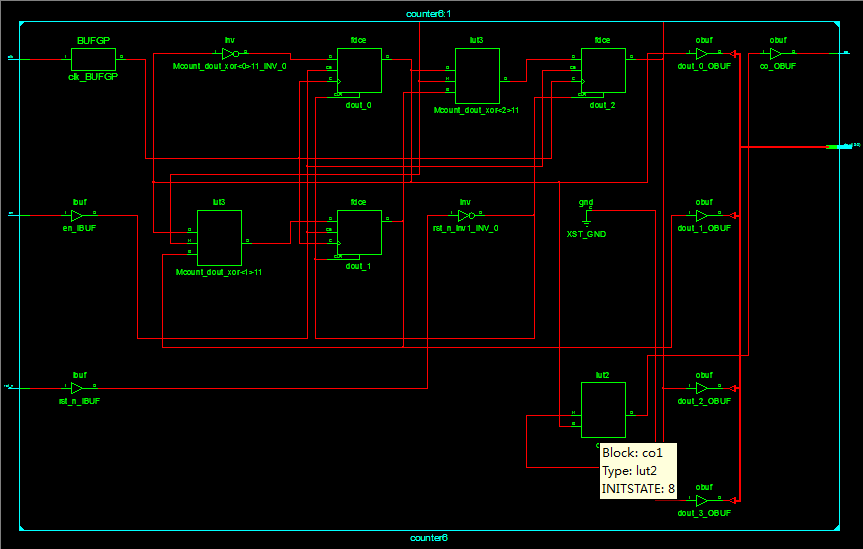## 模10计数器

### Verilog HDL语言描述

``````//模10计数器的Verilog HDL设计
module counter10(clk, rst_n, en, dout, co);

input clk, rst_n, en;
output[3:0] dout;
reg [3:0] dout;
output co;

always@(posedge clk or negedge rst_n)
begin
if(!rst_n)
dout <= 4'b0000;        //系统复位，计数器清零
else if(en)
if(dout == 4'b1001)     //计数值达到5时，计数器清零
dout <= 4'b0000;
else
dout <= dout + 1'b1; //否则，计数器加1
else
dout <= dout;

end

assign co = dout&dout;  //当计数达到5(4'b1001)时，进位为1，计数值为其他，都没有进位

endmodule
``````

### 测试文件

``````//模10计数器的测试文件
`timescale 1ns/1ps
module counter10_tb;

reg clk, rst_n, en;
wire[3:0] dout;
wire co;

//时钟设计周期为2ns
always
begin
#1 clk = ~clk;
end

//初始化
initial
begin
clk = 1'b0;
rst_n = 1'b1;
en = 1'b0;
#2 rst_n = 1'b0;
#2 rst_n = 1'b1; en = 1'b1;    //计数使能信号有效，且不复位

end

counter10 u1(.clk(clk), .rst_n(rst_n), .en(en), .dout(dout), .co(co));

endmodule

``````

### 仿真波形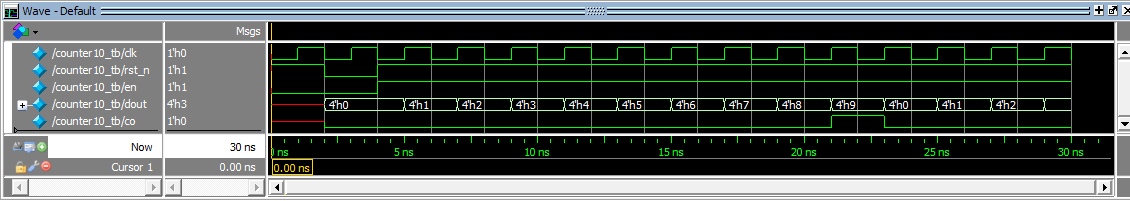### RTL Schematic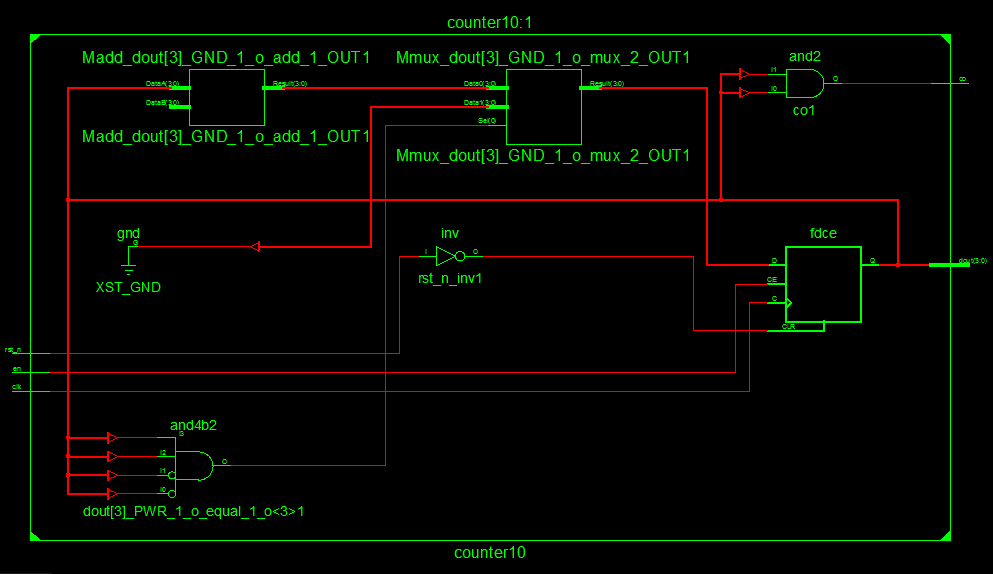### Technology Schematic